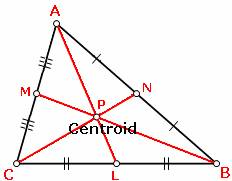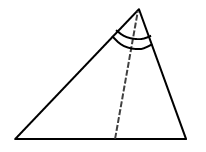# Concurrent lines in Triangles

This set of MCQs helps you brush up on important math topics and prepare you to dive into skill practice.

Start Quiz

[Medians and Centroid]
If AC = 16, what is AM?6

8

10

12

This center is created by the union of three perpendicular bisectors,

Centroid

Incenter

Circumcenter

Orthocenter

The circumcenter is equidistant from each ______________ of the triangle.

Altitude

Vertices

Sides

Median

A segment joining a vertex to the opposite side so that is perpendicular to that side.

Altitude

Median

Perpendicular bisector

Angle bisector

The figure is an example of a(n) .Perpendicular bisector

Angle bisector

Median

Mid segment

The point where three altitude lines are concurrent is called the triangle's :

Centroid

Congruent

Orthocenter

Incenter

When two or more lines intersect at a common point in a plane, then they are called :

Parallel lines

Concurrent lines

Intersecting lines

Skew lines

Quiz/Test Summary
Title: Concurrent lines in Triangles
Questions: 7
Contributed by: